Author: Oscar Cronquist Article last updated on June 10, 2022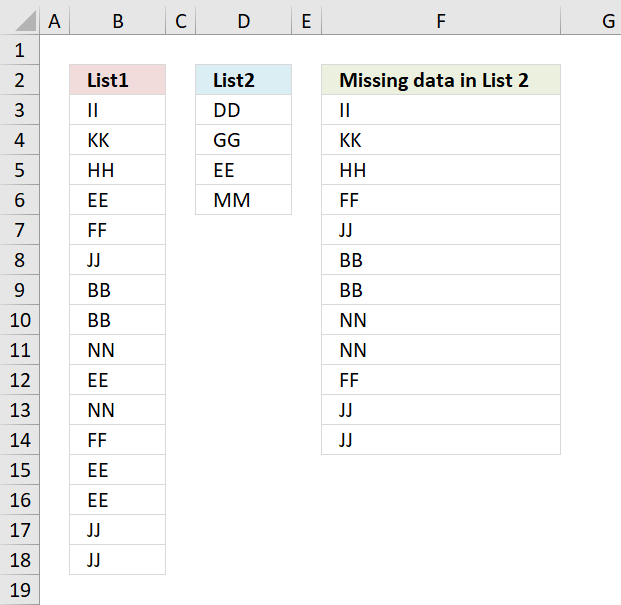This article shows how to compare two nonadjacent cell ranges and extract values that exist only in one of the two columns.

## 1. Extract values that exist only in one of two nonadjacent columnsQuestion: How to filter out data from List 1 that is missing in list 2?

Answer: This formula is useful when comparing two lists to find out what cell values are missing.For instance, inventory comparison.

=INDEX(\$B\$3:\$B\$18, SMALL(IF(ISERROR(MATCH(\$B\$3:\$B\$18, \$D\$3:\$D\$6, 0)), (ROW(\$B\$3:\$B\$18)-MIN(ROW(\$B\$3:\$B\$18))+1), ""), ROWS(\$A\$1:A1)))

How to create an array formula

1. Select cell c2
2. Press with left mouse button on in formula bar
3. Paste above array formula to formula bar4. Press and hold Ctrl + Shift
5. Press Enter
6. Release all keys

You know you have entered an array formula when the formula in the formula bar is surrounded by curly brackets {=array_formula}

### Explaining formula

Step 1 - Compare values between A2:A17 and B2:B5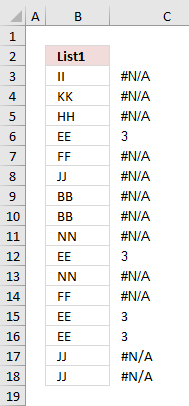The MATCH function looks for a specific value in a cell range or array and returns it's position in that cell range or array.

If the value does not exist in the cell range or array the MATCH function returns #N/A (error value).

MATCH(\$A\$2:\$A\$17, \$B\$2:\$B\$5, 0)

checks if there are any matches. If there are none, an error will occur.

{#N/A,#N/A,#N/A, 3,#N/A,#N/A,#N/A,#N/A,#N/A, 3,#N/A,#N/A, 3, 3,#N/A,#N/A,}

The image to the right shows the array and the corresponding value in column B. It is quite obvious already now which values are missing in the List 2 and which ones that exist.

Step 2 - Identify error values in array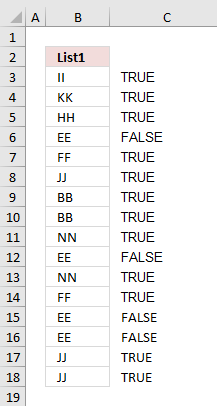If a value in array is an error the ISERROR function returns TRUE else FALSE.

ISERROR(MATCH(\$A\$2:\$A\$17, \$B\$2:\$B\$5, 0))

becomes

ISERROR({#N/A,#N/A,#N/A, 3,#N/A,#N/A,#N/A,#N/A,#N/A, 3,#N/A,#N/A, 3, 3,#N/A,#N/A,})

and returns

{TRUE, TRUE, TRUE, TRUE, FALSE, TRUE, TRUE, TRUE, TRUE, FALSE, TRUE, TRUE, FALSE, FALSE, TRUE, TRUE, }

The array now contains boolean values, TRUE or FALSE.

The IF function in the next step can't handle error values so this step is necessary.

Step 3 - Convert boolean value TRUE to the corresponding row number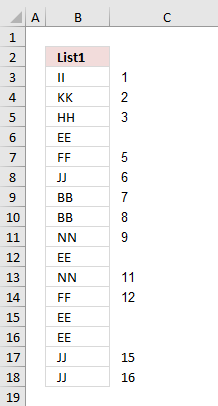The IF function allows you to specify a logical expression and if it evaluates to TRUE one thing happens and if FALSE another thing happens.

IF(ISERROR(MATCH(\$A\$2:\$A\$17, \$B\$2:\$B\$5, 0)), (ROW(\$A\$2:\$A\$17)-MIN(ROW(\$A\$2:\$A\$17))+1), "")

becomes

IF({TRUE, TRUE, TRUE, TRUE, FALSE, TRUE, TRUE, TRUE, TRUE, FALSE, TRUE, TRUE, FALSE, FALSE, TRUE, TRUE, }, {1;2; 3;4;5;6; 7;8;9; 10;11;12; 13;14;15; 16}, "")

and returns {1,2,3,4,"",6,7,8,9,"",11,12,"","",15,16}

If there is an error in the array, replace that error with the row number.

Step 4 - Return the k-th smallest row number

In this example one cell will display one value and in order to do that the small function returns a single row number for each cell allowing you to get a single value in each cell.

SMALL(IF(ISERROR(MATCH(\$A\$2:\$A\$17, \$B\$2:\$B\$5, 0)), (ROW(\$A\$2:\$A\$17)-MIN(ROW(\$A\$2:\$A\$17))+1), ""), ROW(1:1))

becomes

SMALL({1,2,3,4,"",6,7,8,9,"",11,12,"","",15,16}, ROW(1:1))

becomes

SMALL({1,2,3,4,"",6,7,8,9,"",11,12,"","",15,16}, 1)

and returns 1.

Step 5 - Use the row number to get the correct value

The INDEX function allows you to get a value based on a row number and column number.

INDEX(\$A\$2:\$A\$17, SMALL(IF(ISERROR(MATCH(\$A\$2:\$A\$17, \$B\$2:\$B\$5, 0)), (ROW(\$A\$2:\$A\$17)-MIN(ROW(\$A\$2:\$A\$17))+1), ""), ROW(1:1)))

becomes

INDEX(\$A\$2:\$A\$17, 1)

and returns II in cell C2.

## 2. Extract values that exist only in one of two nonadjacent columns - Excel 365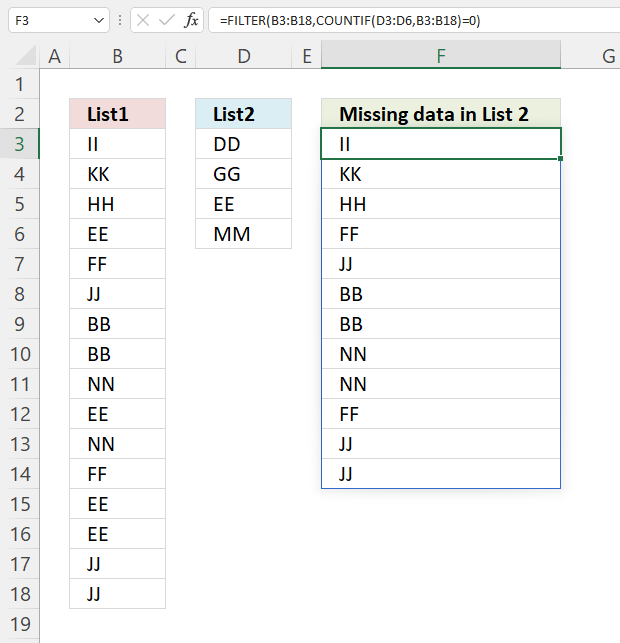The image above demonstrates an Excel 365 formula much smaller than the above, it also extracts missing values between two nonadjacent columns.

Formula in cell F3:

=FILTER(B3:B18, COUNTIF(D3:D6,B3:B18)=0)

### 2.1 Explaining formula

#### Step 1 - Count cells equal to any of the conditions

The COUNTIF function calculates the number of cells that meet a given condition.
COUNTIF(range, criteria)

COUNTIF(D3:D6, B3:B18)

becomes

COUNTIF({"DD"; "GG"; "EE"; "MM"}, {"II"; "KK"; "HH"; "EE"; "FF"; "JJ"; "BB"; "BB"; "NN"; "EE"; "NN"; "FF"; "EE"; "EE"; "JJ"; "JJ"})

and returns

{0; 0; 0; 1; 0; 0; 0; 0; 0; 1; 0; 0; 1; 1; 0; 0}

#### Step 2 - Check if value in the array is equal to 0 (zero)

The equal sign lets you check if a value is equal to another value. The result is a boolean value, TRUE or FALSE.

COUNTIF(D3:D6,B3:B18)=0

becomes

{0; 0; 0; 1; 0; 0; 0; 0; 0; 1; 0; 0; 1; 1; 0; 0}=0

and returns

{TRUE; TRUE; TRUE; FALSE; TRUE; TRUE; TRUE; TRUE; TRUE; FALSE; TRUE; TRUE; FALSE; FALSE; TRUE; TRUE}

#### Step 3 - Filter values based on boolean values

FILTER(B3:B18,COUNTIF(D3:D6,B3:B18)=0)

becomes

FILTER({"II"; "KK"; "HH"; "EE"; "FF"; "JJ"; "BB"; "BB"; "NN"; "EE"; "NN"; "FF"; "EE"; "EE"; "JJ"; "JJ"}, {TRUE; TRUE; TRUE; FALSE; TRUE; TRUE; TRUE; TRUE; TRUE; FALSE; TRUE; TRUE; FALSE; FALSE; TRUE; TRUE})

and returns

{"II"; "KK"; "HH"; "FF"; "JJ"; "BB"; "BB"; "NN"; "NN"; "FF"; "JJ"; "JJ"}.

### Get the Excel filevalues-missing-in-list-1-that-exists-i-list-2v2.xlsx